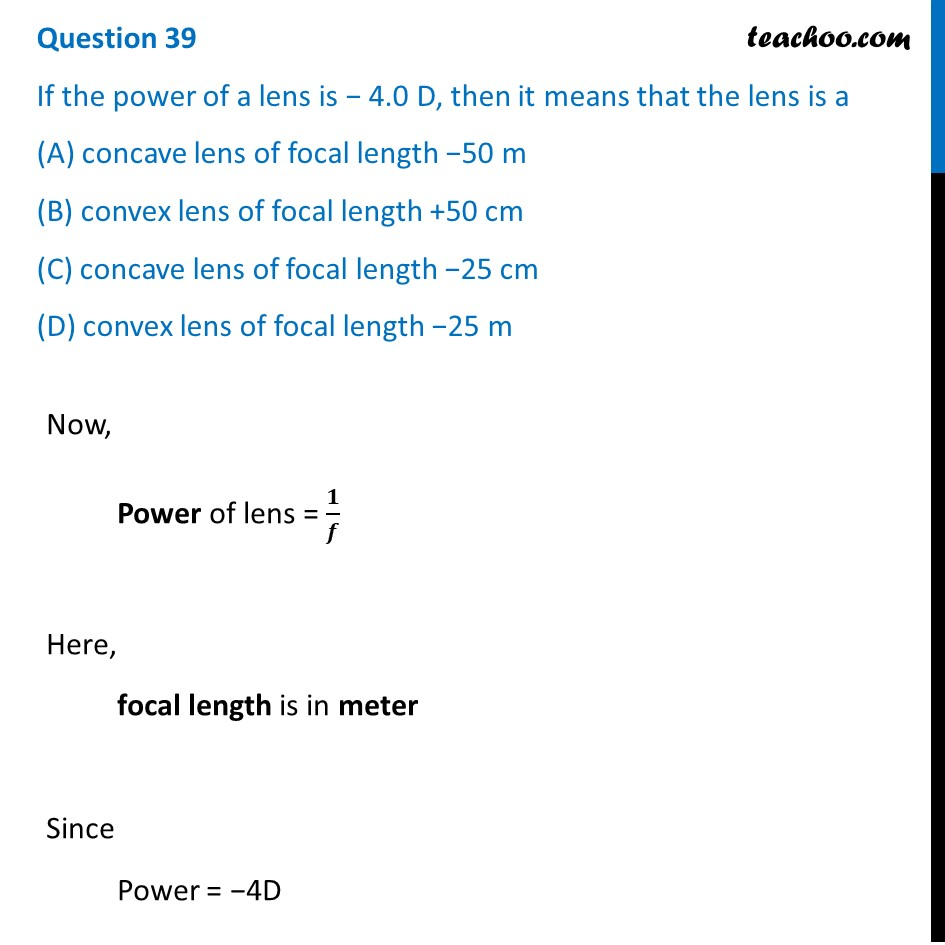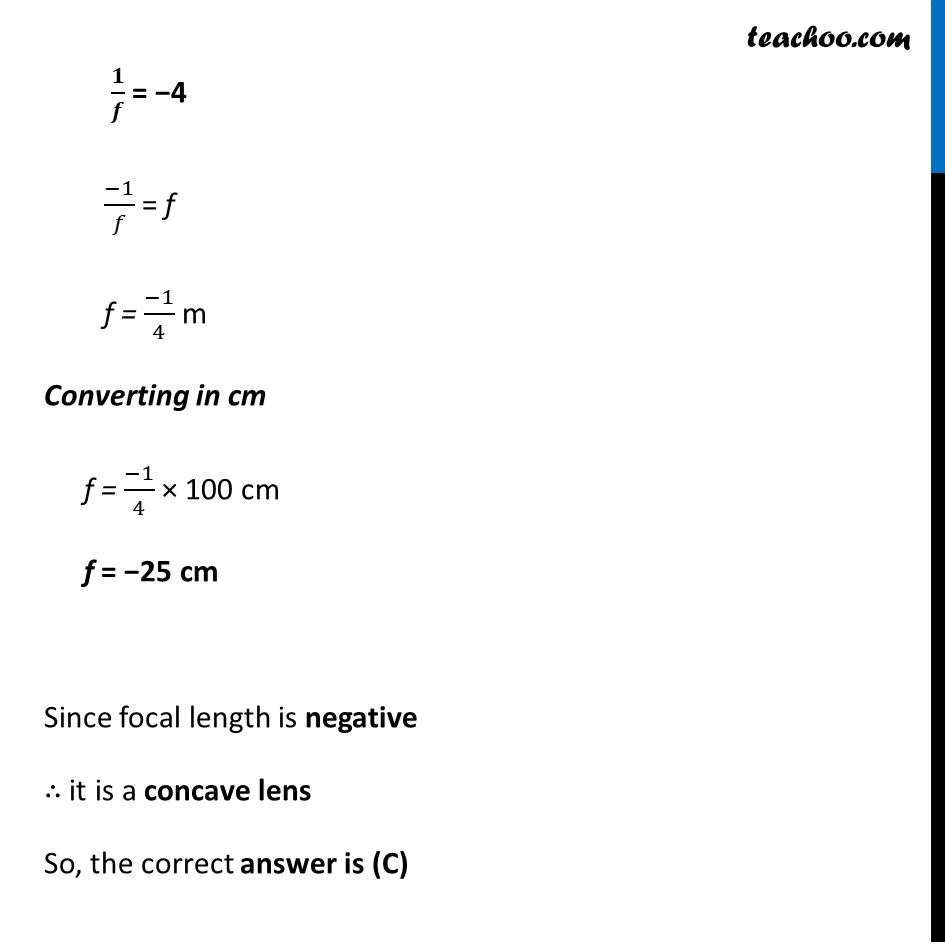Solutions - CBSE Class 10 Sample Paper for 2022 Boards - Science [MCQ]

Class 10
Solutions to CBSE Sample Paper - Science Class 10

## (D) convex lens of focal length -25 mSo, the correct answer is (c)

Learn in your speed, with individual attention - Teachoo Maths 1-on-1 Class

### Transcript

Question 39 If the power of a lens is − 4.0 D, then it means that the lens is a (A) concave lens of focal length −50 m (B) convex lens of focal length +50 cm (C) concave lens of focal length −25 cm (D) convex lens of focal length −25 m Now, Power of lens = 𝟏/𝒇 Here, focal length is in meter Since Power = −4D 𝟏/𝒇 = −4 (−1)/𝑓 = f f = (−1)/4 m Converting in cm f = (−1)/4 × 100 cm f = −25 cm Since focal length is negative ∴ it is a concave lens So, the correct answer is (C)